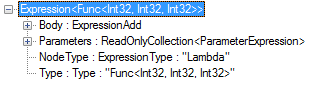# Understanding Expression and Expression Trees

07 Sep 2022
17.9K Views

In .NET, `Expression` is an abstract class which contains static methods and inherited by various types (like ParameterExpression, MethodCallExpression, BinaryExpression etc.) to create expression tree nodes of specific types. Also all these expression-specific expression tree types are defined in `System.Linq.Expressions` namespace.

## Expression Trees

Expression Trees was first introduced in C# 3.0 (Visual Studio 2008), where they were mainly used by LINQ providers. Expression trees represent code in a tree-like format, where each node is an expression (for example, a method call or a binary operation such as x < y). You can also convert expression trees into compiled code and run it. This transformation enables dynamic modification of executable code as well as the execution of LINQ queries in various databases and the creation of dynamic queries.

1. ## Using Expression Lambda

The easiest way to generate an expression tree is to create an instance of the Expression<T> type, where T is a delegate type, and assign a lambda expression to this instance. Let’s take a look at the code.

``` // Create an expression using expression lambda
Expression<Func<int, int, int>> expression = (num1, num2) => num1 + num2;

// Compile the expression
Func<int, int, int> compiledExpression = expression.Compile();

// Execute the expression.
int result = compiledExpression(3, 4); //return 7
```

In this example, the C# compiler generates the expression tree from the provided lambda expression as shown below:

```//Create the expression parameters
ParameterExpression num1 = Expression.Parameter(typeof(int), "num1");
ParameterExpression num2 = Expression.Parameter(typeof(int), "num2");

//Create the expression parameters
ParameterExpression[] parameters = new ParameterExpression[] { num1, num2 };

//Create the expression body

//Create the expression
Expression<Func<int, int, int>> expression = Expression.Lambda<Func<int, int, int>>(body, parameters);
```

This looks much more complicated, but this is what actually happens when you supply a lambda expression to an expression tree.

2. ## Using Expression Tree API

Expression class is used to create expression trees by using the API. In .NET Framework 4, the expression trees API also supports assignments and control flow expressions such as loops, conditional blocks, and try-catch blocks. By using the API, you can create expression trees that are more complex than those that can be created from lambda expressions. Using API, above code can be re-written as:

```//Create the expression parameters
ParameterExpression num1 = Expression.Parameter(typeof(int), "num1");
ParameterExpression num2 = Expression.Parameter(typeof(int), "num2");

//Create the expression parameters
ParameterExpression[] parameters = new ParameterExpression[] { num1, num2 };

//Create the expression body

//Create the expression
Expression<Func<int, int, int>> expression = Expression.Lambda<Func<int, int, int>>(body, parameters);

// Compile the expression
Func<int, int, int> compiledExpression = expression.Compile();

// Execute the expression.
int result = compiledExpression(3, 4); //return 7
```

## Expression Tree Structure

The simple structure of an Expression<TDelegate> has four properties as given below:

1. ## Body

The body of the expression.

2. ## Parameters

The parameters of the lambda expression.

3. ## NodeType

The type of node in the tree

4. ## Type

The type of the expression.## IEnumerable<T> and IQueryable<T> and Expression Trees

In LINQ, a query expression is compiled to expression trees or to delegates, depending on the type that is applied to query result. The IEnumerable<T> type LINQ queries are compiled to delegates and IQueryable or IQueryable<T> queries are compiled to expression trees. In short, LINQ queries that execute in process are compiled into delegates while the queries that executes out of process are compiled into expression trees.

In LINQ, domain-specific queries (like LINQ to SQL, LINQ to Entity) are result into IQueryable<T> type. The C# and Visual Basic compilers compile these queries into code that builds an expression trees at runtime. Then query provider traverse the expression tree and translate it into a query language (like T-SQL) which is appropriate for that data source.

Consider the following LINQ to SQL query expression:

```var query = from c in db.Customers
where c.City == "Delhi"
select new { c.City, c.CompanyName };
```

Here, variable query that is returned by this LINQ query is of type IQueryable. Here is the declaration of IQueryable:

```public interface IQueryable : IEnumerable
{
Type ElementType { get; }
Expression Expression { get; }
IQueryProvider Provider { get; }
}
```

As you can see, IQueryable contains a property of type Expression which holds the expression tree and represents data structure equivalent to the executable code found in a query expression. The IQueryProvider type property hold the LINQ provider (like LINQ to SQL, LINQ to Entity etc.) and based on LINQ provider query is translated into an appropriate query (like T-SQL query). The Type property represents the type of the element(s) that are returned when the expression tree is executed.

In this way, the above code is never actually executed inside your program. It is first translated into the following SQL statement and then executed on SQL Server side.

```SELECT [t0].[City], [t0].[CompanyName]
FROM [dbo].[Customers] AS [t0]
WHERE [t0].[City] = @p0
```

## Expression Trees vs Lambda Expressions

A common misunderstanding is that expression trees are identical to lambda expressions. But this is not true since you can also create and modify expression trees by using API methods i.e. without using lambda expression syntax at all.

Also, every lambda expression cannot be implicitly converted into an expression tree. Only expression lambda is implicitly converted an expression tree and statement lambda i.e. multi-line lambda cannot be implicitly converted into expression tree.